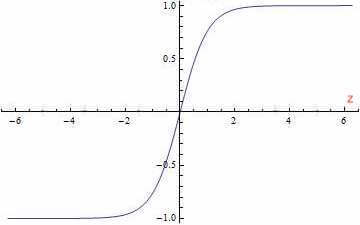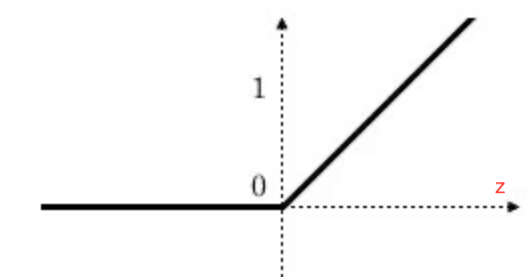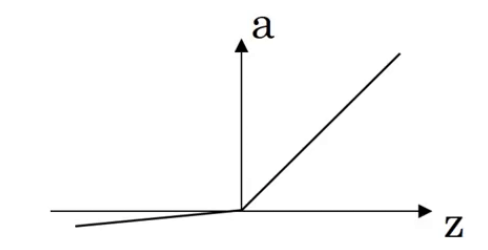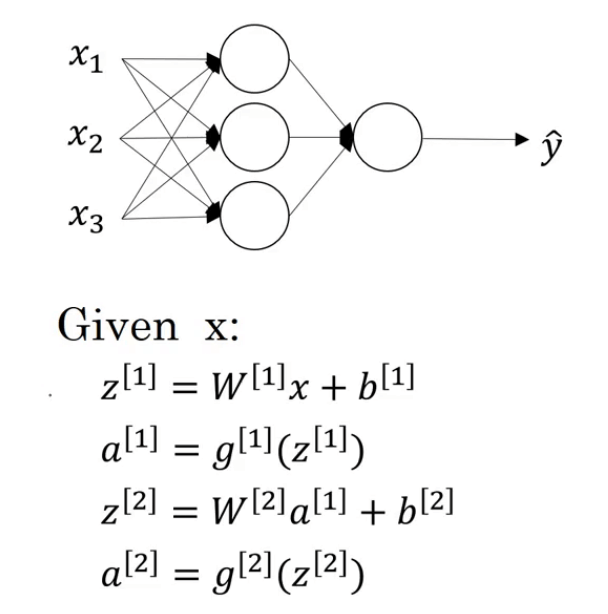# 【深度学习基础】第七课：激活函数

## 激活函数，sigmoid函数，tanh函数，ReLU函数，Leaky ReLU函数，线性激活函数，非线性激活函数

Posted by x-jeff on December 11, 2019

【深度学习基础】系列博客为学习Coursera上吴恩达深度学习课程所做的课程笔记。

# 2.常用的激活函数

## 2.1.tanh函数

tanh函数又叫双曲正切函数$a=g(z)=\tanh (z)=\frac{e^z-e^{-z}}{e^z+e^{-z}}$

a表示activate function，即激活函数。

⚠️tanh函数作为激活函数的效果几乎总是比sigmoid函数的效果要好！但是有一个例外：如果输出的结果为二分类问题，则输出层可以选择sigmoid函数作为激活函数。

❗️不同层的激活函数可以不同。例如我们可以用$g^{}(z^{})$表示第一层的激活函数，用$g^{}(z^{})$表示第二层的激活函数。

⚠️但是tanh函数和sigmoid函数都存在一个很明显的缺点：当z值很大或者很小的时候，函数的斜率就会变得非常小（接近于0），这样会拖慢梯度下降算法。

## 2.2.ReLU函数

ReLU函数(Rectified Linear Unit, ReLU)，又称修正线性单元• 当z为负时，斜率为0。
• z刚好为0时，导数是不存在的。但是在实际情况中，z=0的概率非常非常低。所以在编程实现时，并不需要考虑这个问题。或者也可以当z=0时，把导数值设为0或1。
• 当z为正时，斜率为1。

‼️ReLU函数是大多数神经网络的默认激活函数。因此在不知道选择哪种函数作为激活函数时，可以优先选择ReLU函数。

⚠️ReLU函数的一个缺点就是当z<0时，导数为0。因此引入Leaky ReLU函数克服这个缺点。

## 2.3.Leaky ReLU函数

Leaky ReLU函数的图像见下：⚠️Leaky ReLU的效果通常比ReLU函数要好，但是实际使用的频率并不高。

❗️ReLU函数（或Leaky ReLU函数）不会因为z值过大而导致学习速度减慢，因此以ReLU函数（或Leaky ReLU函数）作为激活函数的神经网络的学习速度通常比sigmoid函数和tanh函数快很多。

# 4.为什么需要非线性激活函数$g^{}$和$g^{}$为非线性激活函数，我们现在将其改为线性激活函数（也称恒等激活函数）：

• $a^{}=z^{}=W^{}x+b^{} \tag{4.1}$
• $a^{}=z^{}=W^{}a^{}+b^{} \tag{4.2}$

\begin{align} a^{} & = W^{}(W^{}x+b^{})+b^{} \\ & = (W^{}W^{})x+(W^{}b^{}+b^{}) \\ & = W'x+b' \\ \end{align}

⚠️可以看出，假设我们使用线性激活函数，那么神经网络的输出其实就是输入特征的线性组合。这种情况下，隐藏层就失去了存在的意义。

❗️只有一个地方可以使用线性激活函数：当解决回归问题时，输出层的激活函数可以为线性激活函数。

# 5.激活函数的导数

1. sigmoid函数：$g’(z)=\frac{d}{dz}g(z)=g(z)(1-g(z))=a(1-a)$。
2. tanh函数：$g’(z)=1-a^2$。
3. ReLU函数：
• $if \ z<0,g’(z)=0$。
• $if \ z>0,g’(z)=1$。
• $if \ z=0,undefined$。但是在实际编码中，可令$g’(z)=1 \ or \ 0$（原因见2.2部分）。
4. Leaky ReLU函数：
• $if \ z<0,g’(z)=0.01$（以2.3部分的公式为例）。
• $if \ z>0,g’(z)=1$。
• 同理，当z=0时，可令$g’(z)=1 \ or \ 0.01$。